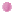Class Notes (from Dr. Ali Muqaibel & Dr. Wajih Abu-Saud):Introduction to Communications  (Ch 1):Introduction to Signals  (Ch 2):Analysis and Transmission of Signals (Ch 3):Amplitude Modulation  (Ch 4):Exponential (Frequency) Modulation  (Ch 5):
• Continuity of FM and PM Signals ( We did not concentrate on continuity, make sure that you can do the two examples and example 5.1 & 5.2 from our textbook)

• Carson's Rule  (Understand the main idea)

• Continue: Carson's Rule (Understand the main idea, you should know the definition of modulation index=deviation ratio for FM , do examples 5.3-5.5 in the textbook)

• FM Receiver  (Sec 5.6 text book) Figure

• Summary of the Chapter (pdf)Sampling &  Pulse Coded Modulation  (Ch 6):Principles of Digital Data Transmission (Ch 7):Matlab Tutorials: